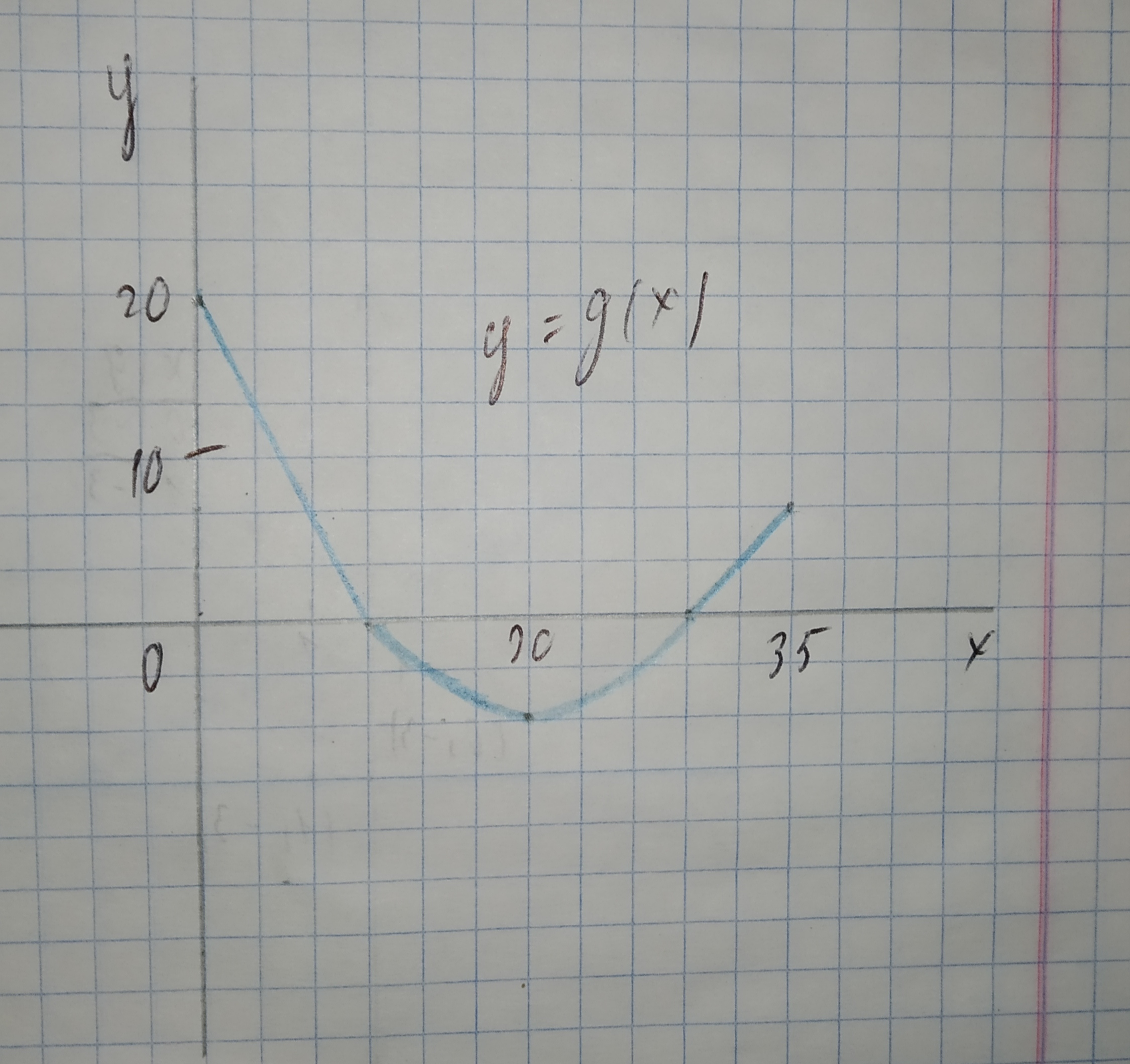# Question# The graph of g consists of two straight lines and a semicircle. Use it to eveluate the integral. 12210203861.jpg int_0^10 g(x)dx

Applications of integrals
ANSWEREDThe graph of g consists of two straight lines and a semicircle. Use it to eveluate the integral.$$\displaystyle{\int_{{0}}^{{10}}}{g{{\left({x}\right)}}}{\left.{d}{x}\right.}$$2021-02-02
The integral $$\displaystyle{\int_{{0}}^{{10}}}{g{{\left({x}\right)}}}{\left.{d}{x}\right.}$$ is the area between the graph of g and the horizontal x-axis on the interval $$\displaystyle{0}\le{x}\le{10}$$.
We note that this are forms a triangle with base b = 10 and height h = 20.
The area of a triangle is the product of the base b and the height h, divided by 2.
$$\displaystyle{\int_{{0}}^{{10}}}{g{{\left({x}\right)}}}{\left.{d}{x}\right.}=\frac{{{b}\cdot{h}}}{{2}}$$
$$\displaystyle=\frac{{{10}\cdot{20}}}{{2}}$$
$$\displaystyle=\frac{{200}}{{2}}$$
=100